Students can download Maths Chapter 6 Trigonometry Ex 6.3 Questions and Answers, Notes, Samacheer Kalvi 10th Maths Guide Pdf helps you to revise the complete Tamilnadu State Board New Syllabus, helps students complete homework assignments and to score high marks in board exams.

## Tamilnadu Samacheer Kalvi 10th Maths Solutions Chapter 6 Trigonometry Ex 6.3

Question 1.
From the top of a rock 50 $$\sqrt { 3 }$$ m high, the angle of depression of a car on the ground is observed to be 30°. Find the distance of the car from the rock.
Let the distance of the car from the rock is “x” m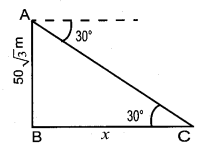In the right ∆ ABC, tan 30° = $$\frac { AB }{ BC }$$
$$\frac{1}{\sqrt{3}}=\frac{50 \sqrt{3}}{x}$$
x = 50 $$\sqrt { 3 }$$ × $$\sqrt { 3 }$$ = 50 × 3
= 150 m
∴ Distance of the car from the rock = 150 mQuestion 2.
The horizontal distance between two buildings is 70 m. The angle of depression of the top of the first building when seen from the top of the second building is 45°. If the height of the second building is 120 m, find the height of the first building.
Let the height of the first building AD be “x” m
∴ EC = 120 – x
In the right ∆ CDE,
tan 45° = $$\frac { CE }{ CD }$$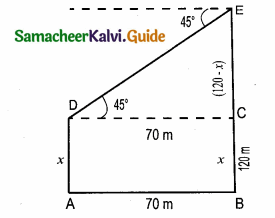1 = $$\frac { 120-x }{ 70 }$$ ⇒ 70 = 120 – x
x = 50 cm
∴ The height of the first building is 50 m

Question 3.
From the top of the tower 60 m high the anles of depression the top and bottom of a vertical lamp post are observed be 38° and 60° respectively
Find the height of the lamp post. (tan 38° = 0.7813,$$\sqrt { 3 }$$ = 1.732)
Let the height of the lamp post be “h”
The height of the tower (BC) = 60 m
∴ EC = 60 – h
Let AB be x
In the right ∆ ABC,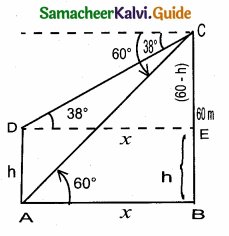tan 60° = $$\frac { BC }{ AB }$$
$$\sqrt { 3 }$$ = $$\frac { 60 }{ x }$$
x = $$\frac{60}{\sqrt{3}}$$ ……..(1)
In the right ∆ DEC, tan 38° = $$\frac { EC }{ DE }$$
0.7813 = $$\frac { 60-h }{ x }$$
x = $$\frac { 60-h }{ 0.7813 }$$ …….(2)
From (1) and (2) we get
$$\frac{60}{\sqrt{3}}$$ = $$\frac { 60-h }{ 0.7813 }$$
60 × 0.7813 = 60 $$\sqrt { 3 }$$ – $$\sqrt { 3 }$$ h
$$\sqrt { 3 }$$ h = 60 $$\sqrt { 3 }$$ – 46.88
= 60 × 1.732 – 46.88
= 103.92 – 46.88
1.732 h = 57.04 ⇒ h = $$\frac { 57.04 }{ 1.732 }$$
h = $$\frac { 570440 }{ 1732 }$$ = 32.93 m
∴ Height of the lamp post = 32.93 mQuestion 4.
An aeroplane at an altitude of 1800 m finds that two boats are sailing towards it in the same direction. The angles of depression of the boats as observed from the aeroplane are
60° and 30° respectively. Find the distance between the two boats. ($$\sqrt { 3 }$$ = 1.732)
C and D are the position of the two boats.
Let the distance between the two boats be “x”
Let BC = y
∴ BD = (x + y)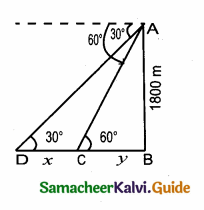In the right ∆ ABC, tan 30° = $$\frac { AB }{ BD }$$
$$\frac{1}{\sqrt{3}}$$ = $$\frac { 1800 }{ x+y }$$
x + y = 1800 $$\sqrt { 3 }$$
y = 1800 $$\sqrt { 3 }$$ – x ……(1)
In the right ∆ ABC, tan 60° = $$\frac { AB }{ BC }$$
$$\sqrt { 3 }$$ = $$\frac { 1800 }{ y }$$
y = $$\frac{1800}{\sqrt{3}}$$ ……….(2)
From (1) and (2) we get
$$\frac{1800}{\sqrt{3}}$$ = 1800 $$\sqrt { 3 }$$ – x
1800 = 1800 × 3 – $$\sqrt { 3 }$$x
$$\sqrt { 3 }$$x = 5400 – 1800
x = $$\frac{3600}{\sqrt{3}}=\frac{3600 \times \sqrt{3}}{\sqrt{3} \times \sqrt{3}}=\frac{3600 \times \sqrt{3}}{3}$$
= 1200 × 1.732 = 2078.4 m
Distance between the two boats = 2078.4 mQuestion 5.
From the top of a lighthouse, the angle of depression of two ships on the opposite sides of it are observed to be 30° and 60°. If the height of the lighthouse is h meters and the line joining the ships passes through the foot of the lighthouse, show that the distance between the ships is $$\frac{4 h}{\sqrt{3}}$$ m.
A and C be the position of two ships.
Let AB be x and BC be y. Distance between the two ships is x + y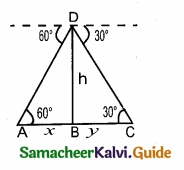In the right ∆ ABD, tan 60° = $$\frac { BD }{ AB }$$
$$\sqrt { 3 }$$ = $$\frac { h }{ x }$$
x = $$\frac{h}{\sqrt{3}}$$ ……(1)
In the right ∆ BCD,
tan 30° = $$\frac { BD }{ BC }$$
$$\frac{1}{\sqrt{3}}$$ = $$\frac { h }{ y }$$
y = $$\sqrt { 3 }$$ h
Distance between the two ships (x + y) = $$\frac{h}{\sqrt{3}}+\sqrt{3} h$$
= $$\frac{h+3 h}{\sqrt{3}}=\frac{4 h}{\sqrt{3}}$$
Hence it is verified

Question 6.
A lift in a building of height 90 feet with transparent glass walls is descending from the top of the building. At the top of the building, the angle of depression to a fountain in the garden is 60°. Two minutes later, the angle of depression reduces to 30°. If the fountain is 30 $$\sqrt { 3 }$$ feet from the entrance of the lift, find the speed of the lift which is descending.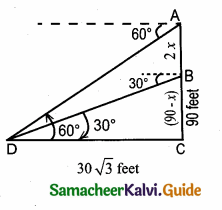tan 30° = $$\frac { BC }{ DC }$$
$$\frac{1}{\sqrt{3}}=\frac{90-2 x}{30 \sqrt{3}}$$
$$\sqrt { 3 }$$ (90 – 2x) = 30$$\sqrt { 3 }$$
(90 – 2x) = $$\frac{30 \sqrt{3}}{\sqrt{3}}$$ ⇒ (90 – 2x) = 30
x = $$\frac { 60 }{ 2 }$$ = 30
Speed of the lift = 30 feet / minute (or) [ $$\frac { 30 }{ 60 }$$ second) 0.5 feet / second